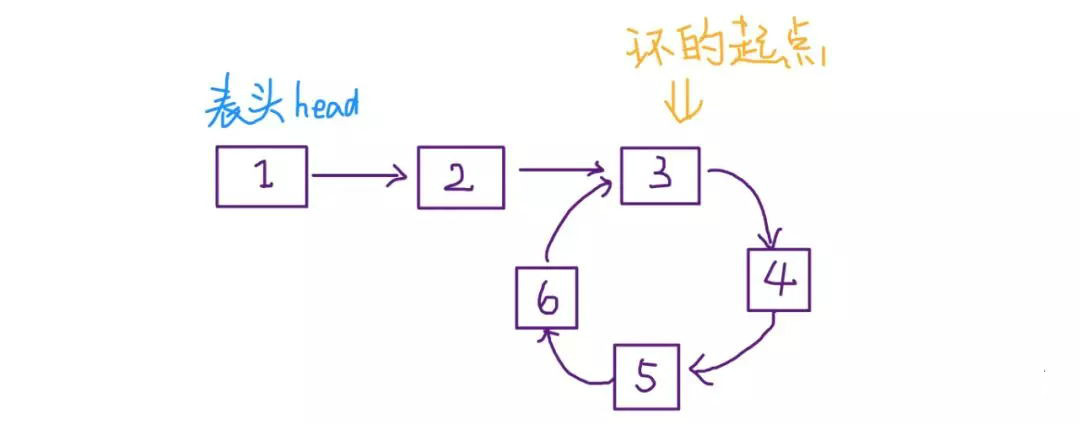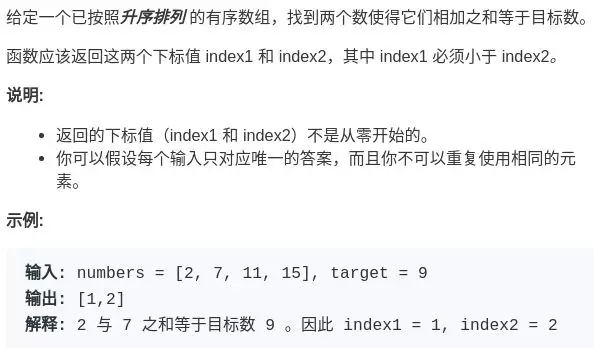## 1.对撞指针(除了二分没啥用)

function fn (list) {
var left = 0;
var right = list.length - 1;

//遍历数组
while (left <= right) {
left++;
// 一些条件判断 和处理
... ...
right--;
}
}

#### 翻转数组

void reverse(int[] nums) {
int left = 0;
int right = nums.length - 1;
while (left < right) {
// swap(nums[left], nums[right])
int temp = nums[left];
nums[left] = nums[right];
nums[right] = temp;
left++;
right--;
}
}

## 2. 快慢指针

#### 判链表环(图的话用这个太慢了)

boolean hasCycle(ListNode head) {
return false;
}

boolean hasCycle(ListNode head) {
ListNode fast, slow;
while(fast != null && fast.next != null) {
fast = fast.next.next;
slow = slow.next;

if (fast == slow)
return true;
}
return false;
}

### 找链表上环的起点这个问题其实不困难，有点类似脑筋急转弯，先直接看代码：

ListNode detectCycle(ListNode head) {
ListNode fast, slow;
while (fast != null && fast.next != null) {
fast = fast.next.next;
slow = slow.next;
if (fast == slow)
break;
}

while (slow != fast) {
fast = fast.next;
slow = slow.next;
}
return slow;
}

### 寻找链表的中点

ListNode slow, fast;
while (fast != null && fast.next != null) {
fast = fast.next.next;
slow = slow.next;
}
// slow 就在中间位置
return slow;

#### 寻找链表的倒数第 k 个元素

ListNode slow, fast;
while (k-- > 0)
fast = fast.next;

while (fast != null) {
slow = slow.next;
fast = fast.next;
}
return slow;

## 3.左右指针

#### 两数之和只要数组有序，就应该想到双指针技巧。这道题的解法有点类似二分查找，通过调节 left 和 right 可以调整 sum 的大小：

#### 滑动窗口算法/尺取法（重要）

9
bcdddbddc

3

#include <iostream>
#include <cstdio>
#include <cstring>
using namespace std;

int l,r,ans,n,ttnum=0,used;
char ss;
int main(){
cin>>n;
scanf("%s",ss);
memset(used,0,sizeof(used));
for(int i=0;i<n;i++){
if(!used[ss[i]-'A']){
ttnum++;
used[ss[i]-'A']=1;
}
}
l=ttnum;r=n;ans=n;
int cnt=0;
while(l<r){
cnt=0;
memset(used,0,sizeof(used));
bool flag=false;
int mid=(l+r)/2;
for(int i=0;i<mid;i++){
if(used[ss[i]-'A']==0){
cnt++;
}
used[ss[i]-'A']++;
if(cnt==ttnum){flag=true;break;}
}
for(int i=1;i<=n-mid;++i){
used[ss[i-1]-'A']--;
if(used[ss[i-1]-'A']==0) cnt--;
if(used[ss[i+mid-1]-'A']==0){
cnt++;
if(cnt==ttnum){
flag=1;
break;
}
}
used[ss[i+mid-1]-'A']++;
}
if(flag){r=mid;ans=mid;}//cout<<"OK @ "<<mid<<endl;}
else {l=mid+1;}//cout<<"ERR @ "<<mid<<endl;}
}
cout<<l;
return 0;
}

-------- 本文结束 感谢阅读 --------微信支付宝